#### STAT 804: 97-3

Assignment 4

Suppose that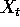is an ARMA(1,1) process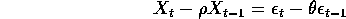1. Suppose we mistakenly fit an AR(1) model (mean 0) to X using the Yule-Walker estimate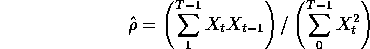In terms of,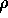and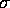what is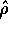close to?

Solution: This is a ratio of two sample covariances. Each converges, as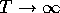to the corresponding theoretical covariance so that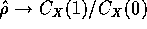. In the previous assignment we computed and found that the lag 1 autocorrelation is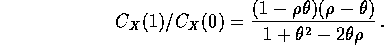This is the limit of.

2. If we use this AR(1) estimateand calculate residuals using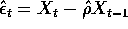what kind of time series is? What will plots of the Autocorrelation and Partial Autocorrelation functions of this residual series look like?

Solution: Let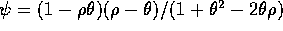denote the autocovariance at lag 1. For large values of T we may write approximately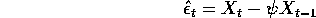or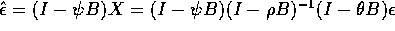or just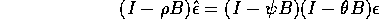which makesan ARMA(1,2) process. By way of answer about the plots I was merely looking for the knowledge that the plots will match those of an ARMA(1,2) with autoregressive parameterand MA parameters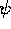and. The model identification problem may well be somewhat harder. It is a useful exercise to generate some data with ar.sim from an ARIMA(1,0,1) and try to model fitting process. Look at what happens if you fit an AR(1) and then look at the residuals; you don't see anything helpful in general.

Richard Lockhart
Mon Dec 8 11:07:38 PST 1997